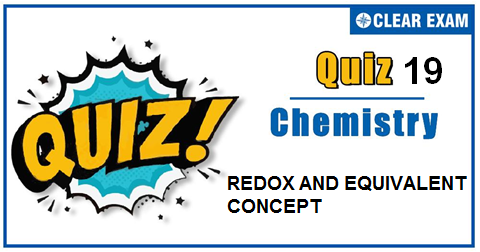## REDOX AND EQUIVALENT CONCEPT QUIZ-19

The one subject in NEET which is candidates who can easily attain good marks is Chemistry. That's the reason, often, one doesn't pay notice and choose to compromise it. But if one wants to rank above others, the tip is to be thorough with NEET chemistry concepts. The understanding of reactions and definite basic understanding is what requires major attention in Chemistry but once done it only gets simpler from there. The main focus on the to-do list should be on getting a hang of the NCERT syllabus of NEET chemistry. .

Q1. In the equation, SnCl2+ 2HgCl2 ⟶Hg2 Cl2+SnCl4. The equivalent weight of stannous chloride (molecular weight = 190) will be :
•  190
•  95
•  47.5
•  154.5
Solution
Sn(2+)⟶Sn(4+)+2e ∴E=M/2=190/2=95

Q2.Which combination is odd with respect to oxidation numbers of S,Cr,N and H respectively:
•  H2 SO5,H2 S2 O8,H2 SO4,SF6
•  K2 Cr2 O7,K2 CrO4,CrO5,CrO2 Cl2
•  NH3,NH4+,N3 H,NO2-
•  CaH2,NaH,LiH,MgH2
Solution
N in NH3,NH4+,N3 H and NO2- has −3, −3, −1/3 and +3 oxidation number respectively.

Q3.  In which case +1 oxidation state is stable than +3?
•   Ga
•  Al
•  Tl
•  B
Solution
Due to inert pair effect which is more predominant in T1.

Q4. Oxidation number of carbon in KCN is :
•  +2
•  -2
•  +1
•  +3
Solution
K − C ≡ N N is more electronegative and thus, has -3 oxidation number as it involves three covalent bonds. Thus, 1+a+(-3)=0 ∴a= +2

Q5.The most stable oxidation state of copper is :
•  +2
•  +1
•  +3
•  +4
Solution
Cu(2+) is more stable than Cu+ although later, has 3d^10configuration. In Cu^+18 electron core is not held properly by nuclear charge and thus, Cu+ is readily converted to Cu(2+).

Q6. The oxidation number of P in KH2 PO2 is :
•  +1
•  +3
• -3
•  +5
Solution
1+2 × (+1)+a+2 × (-2)=0 ∴a= +1

Q7. Which is not an oxidising agent?
•  KClO3
•  O2
•  C6 H12 O6
•  K2 Cr2 O7
Solution
Glucose is reducing agent.

Q8.Oxidation numbers of P in PO4(3-) of S in SO4(2-) and that of Cr in Cr2 O7(2-) are respectively :
•  -3,+6 and+6
•  +5, +6 and +6
•  +3, +6 and +5
•  +5, +3 and +6
Solution
Find oxidation no.in each.

Q9.The stable oxidation states of Mn are∶
•  +2, +3
•  +3, +7
•  +2, +7
•  +3, +5
Solution
Q10. Oxidation number of Mn in K2 MnO4 and MnSO4are respectively:
•  + 7 and +2
•  +6 and +2
•  +5 and +2
• +2 and +6
Solution
Mn has +6 ox.no. in K2 MnO4 and +2 ox.no. in MnSO4.## Want to know more

Please fill in the details below:

## Latest NEET Articles\$type=three\$c=3\$author=hide\$comment=hide\$rm=hide\$date=hide\$snippet=hide

Name

ltr
item
BEST NEET COACHING CENTER | BEST IIT JEE COACHING INSTITUTE | BEST NEET & IIT JEE COACHING: REDOX AND EQUIVALENT CONCEPT QUIZ-19
REDOX AND EQUIVALENT CONCEPT QUIZ-19